# 基于极限载荷与断裂强度的5A06铝合金焊接缺陷安全性评定的数值方法研究Numerical Method Study on Safety Assessment of Welding Defects of 5A06 Aluminum Alloy Based on Ultimate Load and Fracture Strength

• 全文下载: PDF(1072KB)    PP.791-804   DOI: 10.12677/MS.2017.79103
• 下载量: 1,065  浏览量: 1,698   国家科技经费支持

In this paper, a numerical method for assessment the safety of 5A06 aluminum alloy strip with welded defects is established. First, the quantitative relationship between the limit stress and the length of the crack is established by the limit load analysis method, and then the failure assessment curve (FAC) can be obtained. For the 130 mm thick 5A06 Aluminum Alloy butt welding plate, welding residual stress distribution and residual stress intensity factor are obtained by SINTAP, and the stress intensity factor of the working load is calculated by the virtual crack closure tech-nique (VCCT method), then the 5A06 Aluminum Alloy failure assessment diagram (FAD) is estab-lished. The numerical method proposed in this paper can be used to evaluate the safety of the bearing capacity under the condition of a given defect. On the contrary, under the given external load conditions, the limitation of the defect can be determined.

1. 引言

2. 失效评估曲线(FAC)的数值确定方法

2.1. 材料5A06铝合金力学性能参数

5A06铝合金板的应力应变曲线如图1所示  ，相关的力学性能参数如表1所示。

2.2. 有限元模型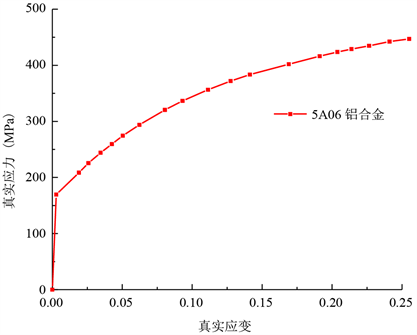Figure 1. Real stress-strain curve of 5A06 aluminum alloyTable 1. Mechanical properties of 5A06 aluminum alloy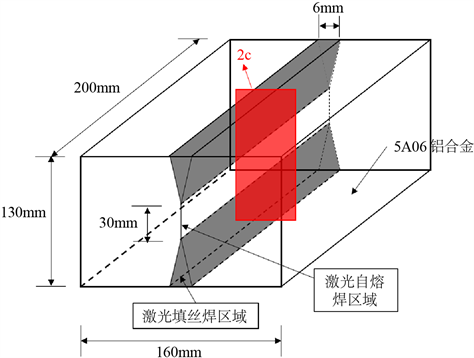Figure 2. 3D model of 5A06 aluminum alloy plate with penetrating crack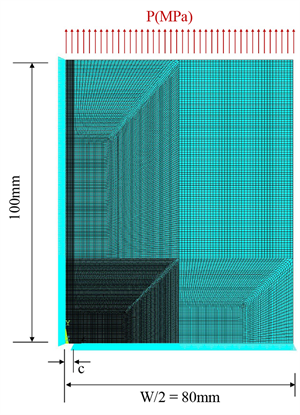Figure 3. Meshing of two dimensional model with central crack

3. 失效评估曲线(FAC)

3.1. 带裂纹5A06铝合金板确定极限应力σNC

$\frac{{\sigma }_{NC}}{{\sigma }_{0}}=0.43+0.4{e}^{20c/W}+0.18{e}^{66.7c/W}$ (1)

3.2. FAC的确定方法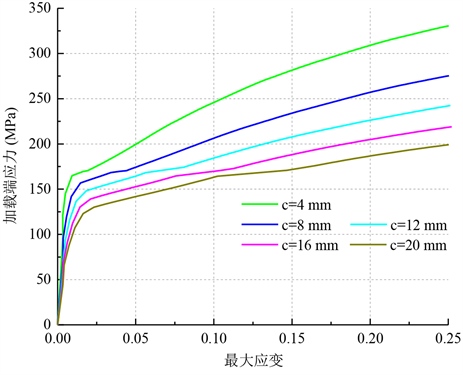Figure 4. Load-end tress and maximum strain curve with different crack lengthsTable 2. Ultimate stress σ N C with different cracks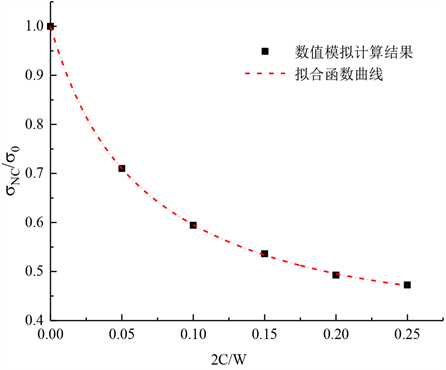Figure 5. The relationship between the limit stress ratio and the dimensionless crack length

${\sigma }_{NC}^{\infty }={\sigma }_{NC}Y$ (2)

$Y=\sqrt{\mathrm{sec}\left(\frac{\pi c}{W}\right)}$ (3)

${K}_{Q}\equiv {\sigma }_{NC}^{\infty }\sqrt{\pi c}$ (4)

${K}_{Q}={K}_{IFM}\left[1-{\delta }_{aci}\left(\frac{{\sigma }_{NC}^{\infty }}{{\sigma }_{0}}\right)\right]\sqrt{1-{\left(\frac{{\sigma }_{NC}^{\infty }}{{\sigma }_{0}}\right)}^{2}}$ (5)

${K}_{Q}=47\left[1-0.2102\left(\frac{{\sigma }_{NC}^{\infty }}{{\sigma }_{0}}\right)\right]\sqrt{1-{\left(\frac{{\sigma }_{NC}^{\infty }}{{\sigma }_{0}}\right)}^{2}}$ (6)Table 3. Fitting value of fracture parameters K I F M and δ a c i of 5A06 aluminum alloy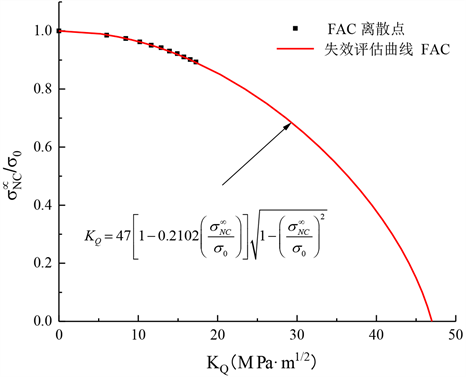Figure 6. Failure assessment curve (FAC) obtained by fitting data points

4.1. 失效评估点(FAP)

$\frac{{\sigma }_{NC}^{\infty }}{{\sigma }_{0}}=\frac{{\sigma }_{app}+{\sigma }_{res}}{{\sigma }_{0}}$ (7)

${K}_{tot}={K}_{app}+{K}_{res}$ (8)

4.1.1. 残余应力的分布与Kres的计算方法

$a=\frac{2c}{2}$$b=\frac{{W}_{1}}{2}$

$a\le b$ 时，

${K}_{res}={\sigma }_{yw}\sqrt{\pi a}$ (9)

$b 时，

${K}_{res}={\sigma }_{yw}\sqrt{\pi a}\left(2/\pi \right)\left\{\left(2/\pi \right)-\frac{\left[{\left({a}^{2}-{b}^{2}\right)}^{1/2}-b\pi /2+b{\mathrm{sin}}^{-1}\left(b/a\right)\right]}{\left({y}_{0}-b\right)}\right\}$ (10)

$a>{y}_{0}$ 时，

${K}_{res}={\sigma }_{yw}\sqrt{\pi a}\left(2/\pi \right)\left\{{\mathrm{sin}}^{-1}\left(b/a\right)-\frac{\left[{\left({a}^{2}-{b}^{2}\right)}^{1/2}-{\left({a}^{2}-{b}^{2}\right)}^{1/2}-b{\mathrm{sin}}^{-1}\left({y}_{0}/a\right)+b{\mathrm{sin}}^{-1}\left(b/a\right)\right]}{\left({y}_{0}-b\right)}\right\}$ (11)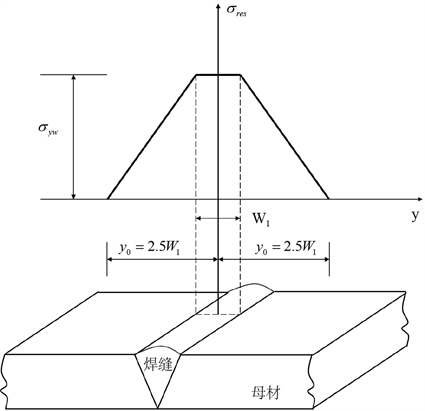Figure 7. Longitudinal welding residual stress distribution diagram

4.1.2. Kapp的计算方法

$W=\frac{1}{2}{\int }_{0}^{\Delta c}u\left(r\right)\sigma \left(r-\Delta c\right)dr$ (12)

$G=\underset{\Delta c\to o}{\mathrm{lim}}\frac{W}{\Delta c}=\underset{\Delta c\to o}{\mathrm{lim}}\frac{1}{2\Delta c}{\int }_{0}^{\Delta c}u\left(r\right)\sigma \left(\Delta c-r\right)\text{d}r$ (13)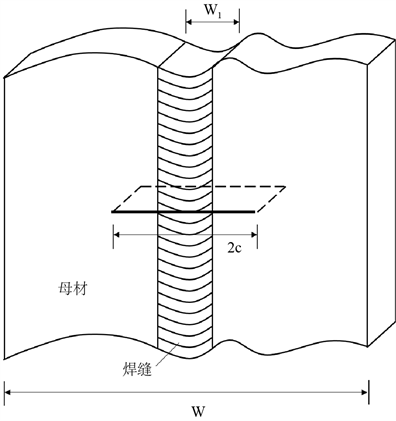Figure 8. Diagrammatic sketch of a plate with a central crack defect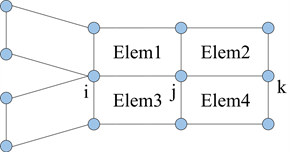Figure 9. Virtual crack closure model

${K}_{app}\text{=}\sqrt{G\text{E}}$ (14)

4.1.3. 5A06铝合金板焊接残余应力分布与Kres的计算

4.1.4. 5A06铝合金板在不同工况下应力强度因子Kapp的计算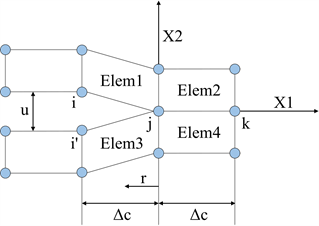Figure 10. The model of crack extension is Δc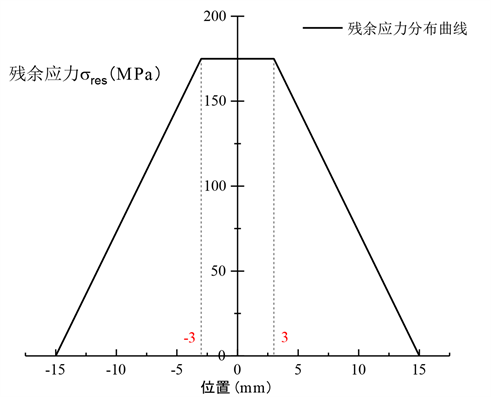Figure 11. Residual stress distribution of 5A06 aluminum alloy

5. 结果与讨论Table 4. Stress intensity factors under different working conditions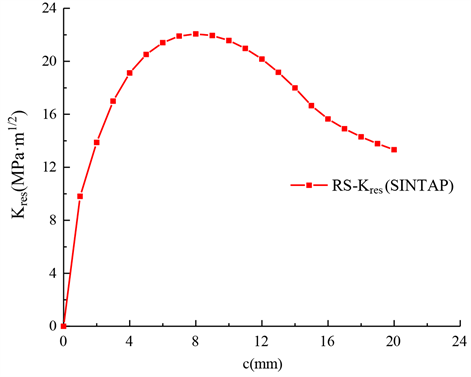Figure 12. The relationship between the residual stress intensity factor and the half crack lengthTable 5. Fracture parameters of 5A06 aluminum alloy with residual stress and working load (40 MPa)Table 6. Fracture parameters of 5A06 aluminum alloy with residual stress and working load (80 MPa and 120 MPa)Figure 13. Failure assessment diagram of 5A06 aluminum alloy

NOTES



*通讯作者。

  Dowling, A.R. and Townley, C.H.A. (1975) The Effect of Defects on Structural Failure: A Two-Criteria Approach. International Journal of Pressure Vessels & Piping, 3, 77-107. https://doi.org/10.1016/0308-0161(75)90014-9  Donzella, G. and Mazzu, A. (2012) Extension to Finite Life of a Failure Assessment Diagram for Contact Fatigue Loading. International Journal of Fatigue, 44, 217-224. https://doi.org/10.1016/j.ijfatigue.2012.04.014  Flom, Y. (2012) Evaluation of Brazed Joints Using Failure Assessment Diagram. In: 5th International Brazing and Soldering Conference.  Ainsworth, R.A. (1993) The Use of a Failure Assessment Diagram for Initiation and Propagation of Defects at High Temperature. Fatigue & Fracture of Engineering Materials & Structures, 16, 1091-1108. https://doi.org/10.1111/j.1460-2695.1993.tb00080.x  Yu, Z., Zheng, Z. and Zhong, Q. (2012) Improved Re-liability Analysis Method Based on the Failure Assessment Diagram. Chinese Journal of Mechanical Engineering, 25, 832-837. https://doi.org/10.3901/CJME.2012.04.832  Zhao, J.P., Huang, W.L. and Dai, S.H. (1997) A New Concept: Probabilistic Failure Assessment Diagram. International Journal of Pressure Vessels & Piping, 71, 165-168. https://doi.org/10.1016/S0308-0161(96)00063-4  Konosu, S. (2009) Assessment Procedure for Multiple Crack like Flaws in Failure Assessment Diagram (FAD). Journal of Pressure Vessel Technology, 131, 041402. https://doi.org/10.1115/1.3122026  Bilby, B.A., Howard, I.C. and Li, Z.H. (1995) Failure Assessment Dia-grams. IV The Inclusion of Constraint Parameters. Proceedings Mathematical & Physical Sciences, 448, 281-291. https://doi.org/10.1098/rspa.1995.0017  Budden, P.J. and Ainsworth, R.A. (2012) The Shape of a Strain-Based Failure Assessment Diagram. International Journal of Pressure Vessels & Piping, 89, 59-66. https://doi.org/10.1016/j.ijpvp.2011.09.004  Ainsworth, R.A., Budden, P.J., Oh, C.Y., et al. (2013) The Treatment of Secondary Strains within a Strain-Based Failure Assessment Diagram. International Journal of Pressure Vessels & Piping, 104, 14-20. https://doi.org/10.1016/j.ijpvp.2013.01.001  Mu, M.Y., Wang, G.Z., Xuan, F.Z., et al. (2017) Fracture As-sessment Based on Unified Constraint Parameter for Pressurized Pipes with Circumferential Surface Cracks. Engineering Fracture Mechanics, 175, 201-218. https://doi.org/10.1016/j.engfracmech.2017.02.004  Budden, P.J., Sharples, J.K. and Dowling, A.R. (2000) The R6 Procedure: Recent Developments and Comparison with Alternative Approaches. International Journal of Pressure Vessels & Piping, 77, 895-903. https://doi.org/10.1016/S0308-0161(01)00012-6  Garwood, S.J., Leggatt, R.H. and Ingham, T. (1997) Structural Collapse, the Failure Assessment Diagram and “Off-the-Shelf” Operation. Fatigue & Fracture of Engineering Materials & Structures, 17, 1418-31. https://doi.org/10.1111/j.1460-2695.1994.tb00785.x  Zerbst, U., Hamann, R. and Beeck, F. (1998) Engi-neering Failure Assessment Methods Applied to Pressurised Components. Nuclear Engineering & Design, 200, 371-382. https://doi.org/10.1016/S0029-5493(00)00252-1  Kanno, S., Hasegawa, K., Shimizu, T., et al. (1992) Analysis of Leak and Break Behavior in a Failure Assessment Diagram for Carbon Steel Pipes. Nuclear Engineering & Design, 138, 251-258. https://doi.org/10.1016/0029-5493(92)90064-3  Kim, Y.J., Shim, D.J., Huh, N.S., et al. (2004) Elastic-Plastic Fracture Mechanics Assessment of Test Data for Circumferential Cracked Pipes. Engineering Fracture Mechanics, 71, 173-191. https://doi.org/10.1016/S0013-7944(03)00095-X  Lee, J.S., Ju, J.B., Jang, J.I., et al. (2004) Weld Crack As-sessments in API X65 Pipeline: Failure Assessment Diagrams with Variations in Representative Mechanical Properties. Materials Science & Engineering A, 373, 122-130. https://doi.org/10.1016/j.msea.2003.12.039  Cravero, S. and Ruggieri, C. (2006) Structural Integrity Analysis of Axially Cracked Pipelines using Conventional and Constraint-Modified Failure Assessment Diagrams. International Journal of Pressure Vessels & Piping, 83, 607-617. https://doi.org/10.1016/j.ijpvp.2006.04.004  Pluvinage, G. (2006) Pipe-Defect Assessment Based on the Limit Analysis, Failure-Assessment Diagram, and Subcritical Crack Growth. Materials Science, 42, 127-139. https://doi.org/10.1007/s11003-006-0065-x  Kamaya, M. and Machida, H. (2010) Reference Stress Method for Evaluation of Failure Assessment Curve of Cracked Pipes in Nuclear Power Plants. International Journal of Pres-sure Vessels & Piping, 87, 66-73. https://doi.org/10.1016/j.ijpvp.2009.11.002  Oyamada, K., Konosu, S. and Ohno, T. (2011) Proposal of Ref-erence Stress for a Surface Flaw on a Cylindrical Component from a Review-with-Comparison of the Local Metal Loss Assessment Rule between API 579-1 and the p-M, Diagram Method. International Journal of Pressure Vessels & Pip-ing, 88, 507-517.  潘建宾, 王国珍, 轩福贞, 等. 核压力容器接管安全端不同焊接结构的失效评定图[J]. 核技术, 2017, 40(5): 82-88.  王元清, 廖小伟, 周晖, 等. 基于SINTAP-FAD方法的含裂纹缺陷钢结构构件安全性评定研究[J]. 工程力学, 2017, 34(5): 42-51.  Kim, Y.J., Kocak, M., Ainsworth, R.A., et al. (2000) SINTAP Defect Assessment Procedure for Strength Mis-Matched Structures. Engineering Fracture Mechanics, 67, 529-546. https://doi.org/10.1016/S0013-7944(00)00072-2  余阳春, 王春明, 余圣甫. 5A06铝合金的激光填丝焊接头组织与性能[J]. 激光技术, 2010, 34(1): 34-36.  王伟年. 不同应力状态5A06铝合金板材断裂预测[D]: [硕士学位论文]. 哈尔滨: 哈尔滨工业大学, 2015.  Kirkwood, M.G., Carmichael, G.D.T. and Moffat, D.G. (1986) Finite Element Stress Analysis of an Equal Diameter Branch Pipe Intersection Subjected to Out-of-Plane and Twisting Moments. Journal of Strain Analysis for Engineering Design, 21, 9-16. https://doi.org/10.1243/03093247V211009  Rajakumar, S. and Christopher, T. (2011) Fracture Strength of Centre Surface Cracked Tensile Specimens Made of 2219-T87 Al Alloy Welding. Chinese Journal of Nonferrous Metals, 21, 2568-2575. https://doi.org/10.1016/S1003-6326(11)61093-5  王生楠, 于红艳. 含裂纹结构的极限载荷分析[J]. 机械科学与技术, 2004, 23(7): 863-865.  Leggate, R.H. and Sanderson, R.M. (1999) Stress Intensity Due to Residual Stresses. HSC Publications.  解德, 钱勤, 李长安. 断裂力学中的数值计算方法及工程应用[M]. 北京: 科学出版社, 2009.  Sung, P.H., Yang, W.H. and Kim, C. (2002) Stress Intensity Factors for Elliptical Arc through Cracks in Mechanical Joints by Virtual Crack Closure Technique. KSME International Journal, 16, 182-191. https://doi.org/10.1007/BF03185169